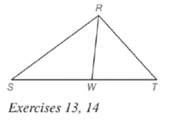Chapter 5.6, Problem 13E### Elementary Geometry for College St...

6th Edition
Daniel C. Alexander + 1 other
ISBN: 9781285195698

#### Solutions

Chapter
Section### Elementary Geometry for College St...

6th Edition
Daniel C. Alexander + 1 other
ISBN: 9781285195698
Textbook Problem
5 views

# Given: R W → bisects ∠ S R T Do the following equalities hold? a) S W = W T b) R S R T = S W W TTo determine

a)

To check: Whether the equality SW=WT holds or not.

Explanation

Given:

RW bisects SRT.

Theorem used:

Angle bisector theorem:

If a ray bisects one angle of a triangle, then it divides the opposite side into segments whose lengths are proportional to the lengths of the two sides that form the bisected angle

To determine

b)

To check: Whether the equality RSRT=SWWT holds or not.

### Still sussing out bartleby?

Check out a sample textbook solution.

See a sample solution

#### The Solution to Your Study Problems

Bartleby provides explanations to thousands of textbook problems written by our experts, many with advanced degrees!

Get Started

#### In Problems 15-28, perform the operations if possible. 24. .

Mathematical Applications for the Management, Life, and Social Sciences

#### Simplify the expressions in Exercises 97106. 21/32122/321/3

Finite Mathematics and Applied Calculus (MindTap Course List)

#### In Exercises 107-120, factor each expression completely. 120. x3 27

Applied Calculus for the Managerial, Life, and Social Sciences: A Brief Approach

#### Solve each inequality. x2

Trigonometry (MindTap Course List)

#### Solve each equation. x42x2+1=0

College Algebra (MindTap Course List)